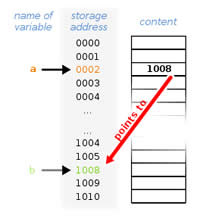# Difference Between Integer and Pointer

##### Key Difference: Integers refer to any data type that represents a subset of the mathematical integers. Pointers are data types that whose value refers to another value or data stored in the system.

Integer and Pointers are both are commonly used in computer programming languages. Both the terms are different from each other but refer to data that is stored in the computer. Integers refer to any data type that represents a subset of the mathematical integers. Pointers are data types that whose value refers to another value or data stored in the system.An ‘integer’ is a datum of integral data type which represents a subset of mathematical integers. These data types may or may not contain negative values and has different sizes. An integer is often considered as a primitive data type in computer languages and can only represent a subset of all integers, since practical computers are of finite capacity. Integers are usually represented in binary digits.

The integral types may be signed or unsigned; signed means that it can represent both positive and negative integers, while unsigned means that it can only represent non-negative integers. The value of an integral data type is the mathematical integer that it corresponds to. The value of an integer is specified in the source of the code in a sequence of digits without any separators or spaces, but can be occasionally prefixed with a + or -.  The negative numbers can be represented in three ways; by one’s complement, two’s complement or sign-magnitudes.

However, unlike mathematical integers, a typical integral data has a maximum and a minimum value. The maximum is called MAXINT or, as in the C standard library limits.h header, INT_MAX. Various different CPUs use different types of integral data.In computing, a pointer is a variable that holds the address of a core storage location. It is a data type whose value points directly to another value that is stored in the computer’s memory.

For high-level programming languages, pointers effectively take the place of general purpose registers in low-level languages such as assembly language or machine code, but may be in available memory. A pointer refers to a location in memory, and obtaining the value at the location a pointer refers to is known as dereferencing the pointer.

Points are most commonly used to improve performance for repetitive operations such as traversing strings, lookup tables, control tables and tree structures. Pointers can allow protected as well as unprotected admission to the memory addresses and can be used to hold addresses of entry points. It is also a much easier and a faster option to copy and dereference the pointers than to continuously keep copying and accessing the data that the pointer is pointing to. The value of a pointer determines which memory address is to be used in a calculation. Pointers are usually stated as a fundamental data type in programming languages.

Image Courtesy: ehowcdn.com, wikimedia.org